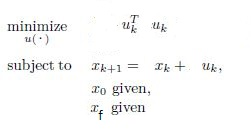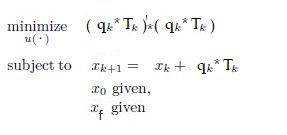# Constrained Discrete-time optimal control(two boundary value problem)

Hi, I’m a student and this is my first approach to optimization and obviously the same for cvx . I trying to develop a guidance control algorithm and it works if I consider a simple optimization problem as follow(it works well on 4°order system built on the same philosophy but i will use 1° order system for to explain my problem in a simple way ):I rewrite my system in such a way to eliminate the dependence to x(k)

% x(2:k+1)=Fxo+Hu =

% x[2:k+1] =[A … A^k]'x0 + [ A^0B 0 … 0 ]
% %%%%%%%%%%%%%[ A^1*B B 0 0 ]U_vett(1:K)
% %%%%%%%%%%%%%[ … … B 0 ]
%%%%%%%%%%%%%%[ A^k
B A^(k-1)B A^(k-2)B A^(k-n)B ]

% with A=1 B=1

clear all
clc

N=3;
%x(2:k+1)=Fxo+Hu
F=[1;1;1];
H=[1 0 0;1 1 0;1 1 1];

cvx_begin

variables u(N) v(N)

minimize( u’*u)

``````subject to
``````

v==Fv(1)+Hu*0.1;

``````-10<=u<=10
v(1)==0;
v(N)==1;
``````

cvx_end

figure(1)
hold on
plot(v,‘b’)
plot(u,‘r’)

BUT if I consider the optimization problem as follow :
.It doesn’t work and the causes is q.T(element-wise multiplication) and i don’t understand why. q.T is a column vector as u in the previous example. The . is supported in CVX as written on reference guide–>Arithmetic operators
the code is the following:
clear all
clc

N=3;

cvx_begin

variables q(N) T(N) v(N)

minimize( (q.T)’(q.*T)+v’*v)

``````subject to
``````

v==[1;1;1]*v(1)+[1 0 0;1 1 0;1 1 1]*q.T0.1;
-1<=T<=1
-1<=q<=1
v(1)==0;
v(N)==10;
cvx_end

%Error using .* (line 262)
%Disciplined convex programming error:
%Invalid quadratic form(s): not a square.

I had tried to create a function inside CVX but don’t work.

I hope that someone could help me. I would be very very gratefull

`q.*T` where `q` and `T` are both CVX variables is not in compliance with the DCP rules. Of course, if you declare a CVX varaible `u`, and have it be what you think of as `q.*T`, but CVX is never presented with `q.*T`, then you have gotten around that.

DCP doesn’t allow multiplication of 2 or more different CVX variables, except in geometric programming mode. Consider `x*y` where `x` and `y` are scalar CVX variables. That is an indefinite (non-convex) form. Things don’t get better in higher dimensions. Of course if you don’t need `x` and `y`, other than as their product, then you can get around that as above.

houston we have a problem… I need x, y in higher dimensions. Thanks mark you saved me from becoming crazy !!!